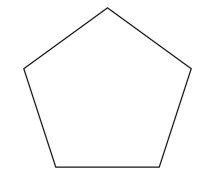# Swift Program to Calculate Area of Pentagon

This tutorial will discuss how to write swift program to calculate area of pentagon.

A pentagon is a two-dimensional shape with 5 sides. It also contains five interior angles and their sum is 540 degrees. It is also known as five side polygon. The total amount of space enclosed inside the five sides of the pentagon is known as the area of the pentagon.## Formula

Following is the formula of the area of the pentagon:

Area = 1/4(sqrt(5(5+2√5))q2)


Below is a demonstration of the same −

Input

Suppose our given input is −

side = 6


Output

The desired output would be −

Area of the pentagon= 61.93718642120281


## Algorithm

Following is the algorithm −

Step 1- Create a function with return value.

Step 2- Find the area of the pentagon using the following formula:

return (sqrt(5*(5+2*sqrt(5))) * q * q)/4


Step 3- Calling the function and pass the side in the function as a parameter.

Step 4- Print the output.

## Example

The following program shows how to calculate the area of pentagon.

import Foundation
import Glibc

// Creating a function to find the area of pentagon
func pentagonArea(q:Double) -> Double{
return (sqrt(5*(5+2*sqrt(5))) * q * q)/4
}
var num = 10.0
print("Length of the side is", num)
print("Area of the pentagon:", pentagonArea(q:num))


## Output

Length of the side is 10.0
Area of the pentagon: 172.0477400588967


Here, in the above program we create a function which return the area of the pentagon using the following formula −

return (sqrt(5*(5+2*sqrt(5))) * q * q)/4


Here, we use sqrt() function to find the square root.

Updated on: 30-Nov-2022

121 Views